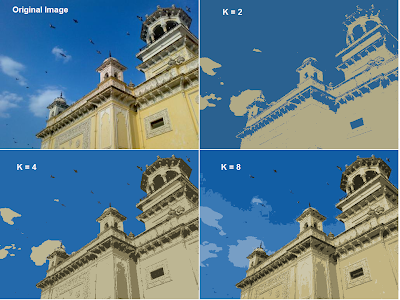## Sunday, January 27, 2013

### K-Means Clustering - 3 : Working with OpenCV

Hi,

In the previous articles, K-Means Clustering - 1 : Basic Understanding and K-Means Clustering - 2 : Working with Scipy, we have seen what is K-Means and how to use it to cluster the data. In this article, We will see how we can use K-Means function in OpenCV for K-Means clustering.

OpenCV documentation for K-Means clustering : cv2.KMeans()

Function parameters :

Input parameters :

1 - samples : It should be of np.float32 data type, and as said in previous article, each feature should be put in a single column.

2 - nclusters(K
) : Number of clusters

3 - criteria : It is the algorithm termination criteria. Actually, it should be a tuple of 3 parameters. They are ( type, max_iter, epsilon ):
3.a - type of termination criteria : It has 3 flags as below:
- cv2.TERM_CRITERIA_EPS - stop the algorithm iteration if specified accuracy, epsilon, is reached.
- cv2.TERM_CRITERIA_MAX_ITER - stop the algorithm after the specified number of iterations, max_iter.
- cv2.TERM_CRITERIA_EPS + cv2.TERM_CRITERIA_MAX_ITER - stop the iteration when any of the above condition is met.

3.b - max_iter - An integer specifying maximum number of iterations.
3.c - epsilon - Required accuracy

4 - attempts : Flag to specify the number of times the algorithm is executed using different initial labellings. The algorithm returns the labels that yield the best compactness. This compactness is returned as output.

5 - flags : This flag is used to specify how initial centers are taken. Normally two flags are used for this : cv2.KMEANS_PP_CENTERS and cv2.KMEANS_RANDOM_CENTERS. (I didn't find any difference in their results, so I don't know where they are suitable. For time-being, I use second one in my examples).

Output parameters:

1 - compactness : It is the sum of squared distance from each point to their corresponding centers.

2 - labels : This is the label array (same as 'code' in previous article) where each element marked '0', '1'.....

3 - centers : This is array of centers of clusters.

Now let's do the same examples we did in last article. Remember, we used random number generator to generate data, so data may be different this time.

1 - Data with Only One Feature:

Below is the code, I have commented on important parts.

import numpy as np
import cv2
from matplotlib import pyplot as plt

x = np.random.randint(25,100,25)
y = np.random.randint(175,255,25)
z = np.hstack((x,y))
z = z.reshape((50,1))

# data should be np.float32 type
z = np.float32(z)

# Define criteria = ( type, max_iter = 10 , epsilon = 1.0 )
criteria = (cv2.TERM_CRITERIA_EPS + cv2.TERM_CRITERIA_MAX_ITER, 10, 1.0)

# Apply KMeans
ret,labels,centers = cv2.kmeans(z,2,criteria,10,cv2.KMEANS_RANDOM_CENTERS)

# Now split the data depending on their labels
A = z[labels==0]
B = z[labels==1]

# Now plot 'A' in red, 'B' in blue, 'centers' in yellow
plt.hist(A,256,[0,256],color = 'r')
plt.hist(B,256,[0,256],color = 'b')
plt.hist(centers,32,[0,256],color = 'y')
plt.show()


Below is the output we get :KMeans() with one feature set

2 - Data with more than one feature :

Directly moving to the code:

import numpy as np
import cv2
from matplotlib import pyplot as plt

X = np.random.randint(25,50,(25,2))
Y = np.random.randint(60,85,(25,2))
Z = np.vstack((X,Y))

# convert to np.float32
Z = np.float32(Z)

# define criteria and apply kmeans()
criteria = (cv2.TERM_CRITERIA_EPS + cv2.TERM_CRITERIA_MAX_ITER, 10, 1.0)
ret,label,center = cv2.kmeans(Z,2,criteria,10,cv2.KMEANS_RANDOM_CENTERS)

# Now separate the data, Note the flatten()
A = Z[label.flatten()==0]
B = Z[label.flatten()==1]

# Plot the data
plt.scatter(A[:,0],A[:,1])
plt.scatter(B[:,0],B[:,1],c = 'r')
plt.scatter(center[:,0],center[:,1],s = 80,c = 'y', marker = 's')
plt.xlabel('Height'),plt.ylabel('Weight')
plt.show()


Note that, while separating data to A and B, we used label.flatten(). It is because 'label' returned by the OpenCV is a column vector. Actually, we needed a plain array. In Scipy, we get 'label' as plain array, so we don't need the flatten() there in Scipy. To understand more, check the 'label' in both the cases.

Below is the output we get :KMeans() with two feature sets

3 - Color Quantization :

import numpy as np
import cv2
from matplotlib import pyplot as plt

Z = img.reshape((-1,3))

# convert to np.float32
Z = np.float32(Z)

# define criteria, number of clusters(K) and apply kmeans()
criteria = (cv2.TERM_CRITERIA_EPS + cv2.TERM_CRITERIA_MAX_ITER, 10, 1.0)
K = 8
ret,label,center = cv2.kmeans(Z,K,criteria,10,cv2.KMEANS_RANDOM_CENTERS)

# Now convert back into uint8, and make original image
center = np.uint8(center)
res = center[label.flatten()]
res2 = res.reshape((img.shape))

cv2.imshow('res2',res2)
cv2.waitKey(0)
cv2.destroyAllWindows()


Below is the output we get :Color Quantization with KMeans Clustering

Summary :

So finally, We have seen how to use KMeans clustering with OpenCV. I know, I haven't explained much in this article, because it is same as the previous article. Just a function is changed.

So this series on KMeans Clustering algorithm ends here.

Feel free to post your comments, feedback...

Feel free to share it with your friends....

Regards,
Abid Rahman K.

1.2.